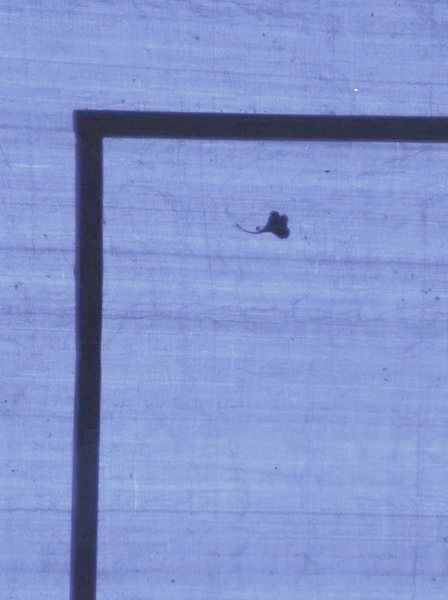explorations, interests & opinions of a mind

Two problems from Google treasure hunt, which although not quite tough, but nevertheless were interesting.

One was about a robot starting from top left corner of a matrix, trying to reach the bottom right corner. Robot could only go right or down. The problem was to find the number of unique possible paths. My first thought was to use dynamic programming. Some more thoughts, and I was able to see a pattern in the solution. The basic idea was, like in dynamic programming, to solve the smaller problems first. The observation was that the paths could be calculated one level at a time. The paths are calculated along a line parallel to the anti-diagonal, as it sweeps through the matrix from destination cell towards the starting cell.

Here's the code doing just that.

int main(int argc, char ** argv) {  int r, c, i, j, diag;  unsigned long long *pa;  assert(argc == 3);
r = atoi(argv);  c = atoi(argv);
diag = r < c ? r : c;  pa = calloc( diag, sizeof pa );
for (i=0;i<diag;i++)    pa[i] = 0;  pa = 1;
for (i=0;i<r+c-2;i++)    for (j=diag-1;j>0;j--)      pa[j] = pa[j] + pa[j-1];
printf("paths %llu \n", pa[diag-1]);
return 0;}

The implementation worked for small inputs. But for the actual input, long long was not enough (and assumably intended by the problem designer). Some more thoughts about the process, and the insight came. The pattern seemed to be familiar, although incomplete. It was the Pascal's triangle . It was easy to find out the entry needed from the Pascal's triangle and its corresponding value ($\mathrm{C}^{n+m-2}_{n-1}$). But still, to calculate it, would need more precision than whats available with C data types (otherwise my program would have given the answer). Google also didn't help. Looking for bignum library, lead me to this expression calculator. All I had to do was fill in the equation and copy paste the solution.

The other one was the last problem. Here is a verbatim instance of the problem.

Find the smallest number that can be expressed as the sum of 3 consecutive prime numbers, the sum of 35 consecutive prime numbers, the sum of 553 consecutive prime numbers, the sum of 829 consecutive prime numbers, and is itself a prime number. For example, 41 is the smallest prime number that can be expressed as the sum of 3 consecutive primes (11 + 13 + 17 = 41) and the sum of 6 consecutive primes (2 + 3 + 5 + 7 + 11 + 13 = 41).

The smaller sequence would always end with larger primes, than the longer sequence. Reason being that the same sum is distributed in a smaller set of consecutive primes. How does that help? Slide the smallest sequence to include the next prime, and simultaneously, update all later sequences to have sum greater than or equal to sequences smaller than them. Here's a little cryptic code for the solution.

struct cseq {  int seql;			/* seq len */  int seqt;			/* seq top */  int seqs;			/* seq sum */} *sa;
void slide_sequence(struct cseq *s) {  s->seqt++;  s->seqs -= prime[s->seqt - s->seql];  s->seqs += prime[s->seqt];}
int align_to_sum(struct cseq *s, int sum) {  while (s->seqs < sum) slide_sequence(s);
return s->seqs == sum;}
int main() {  /* initialize sa and primes array and other variables. */
for each consecutive prime {    /* invar: bigger sequences have sum >= smaller once. */    eqcnt=0;
slide_sequence( &(sa) );
for (j=1;j<scnt;j++)      if (align_to_sum(&(sa[j]), sa.seqs))        eqcnt++;
if (eqcnt == scnt-1) {      if (isprime(sa.seqs) {        print_sequence(sa, scnt);        break;      } else {        continue;      }    }  }}

I used an already generated list of primes, from here .

Here are the timing details.

\$time ./a.outseq:     3, start: 221709, end: 221711, sum: 9221231seq:    35, start: 23088, end: 23122, sum: 9221231seq:   553, start:  1650, end:  2202, sum: 9221231seq:   829, start:   930, end:  1758, sum: 9221231
real   0m0.210suser   0m0.208ssys    0m0.000s
...